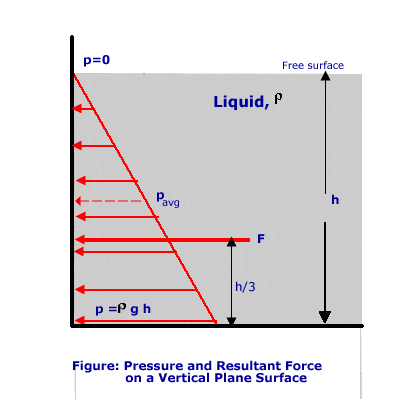# Average pressure

I need to work out average pressure in order to work out horizontal thrust on an oil tank. I know that pressure=density*height*acceleration, although I want to know what 'top' and 'bottom' surfaces refer to. There is a tank filled with liquid, to a given depth and the height of the tank is given. So how do I work out the average pressure? Do I just sub in the to heights? The depth of the liquid and the height of the tank?

Thanks.

I meant add them together and divide by to, not sub in two pgh.

Mech_Engineer
Gold Member
Since the pressure linearly increases with height, and the pressure at the top of the fluid is zero, the average pressure is half the maximum pressure (see link). The resultant force occurs at 1/3 the height of the tank, acting at the centroid of the "force triangle" which describes the pressure as a function of height.

http://www.savannahstate.edu/cost/eng-tech/faculty/jayjan2010/jay/hehslidec2.htm

SavannahState.edu said:
The pressure will vary from zero at the surface to (r g h) at the bottom of the wall. The pressure distribution (varying linearly with depth) will be as shown in figure above.

The total resultant force per unit length of wall = average pressure x area = pavg x h x 1

Average pressure = (r g h)/2

Therefore, Resultant force = F = (r g h)/2 x h x 1 = 1/2 {r g h2}

The resultant force acts perpendicular to the wall at the centroid of the pressure distribution triangle, at a distance of h/3 from the bottom of the wall.